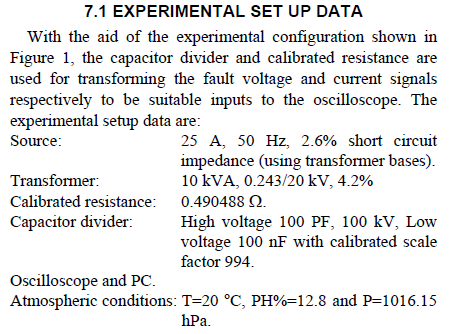# Modeling and Experimental Verification of High Impedance Arcing Fault in Medium Voltage Networks（中压电网高阻抗电弧故障的建模与实验验证）

## 3. Arc Modeling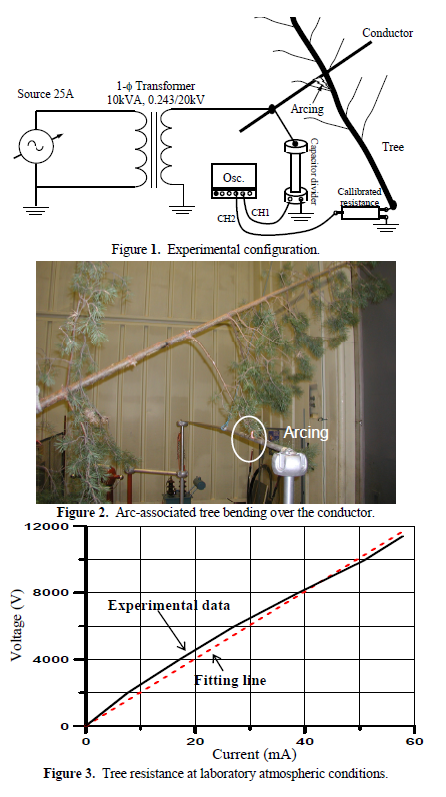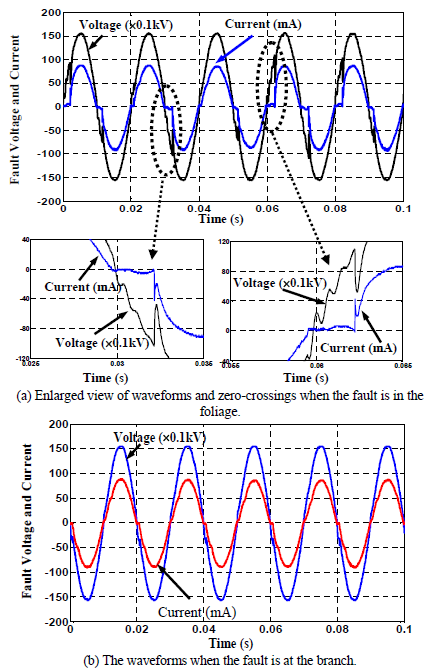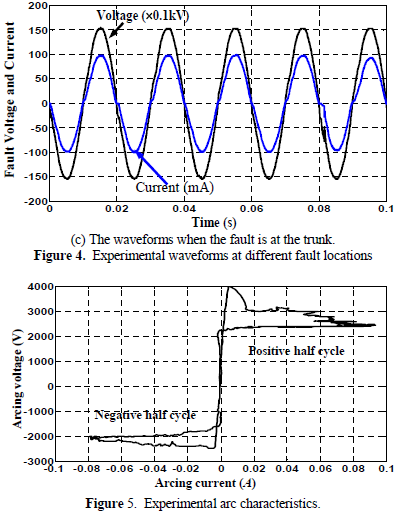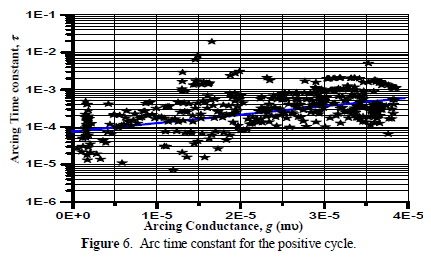$\frac = \frac{1}{\tau }(G - {\text{g}})\tag{1}$
 其中g是时变电弧电导，G = i / Varc是固定电弧电导， i 是弧电流的绝对值，Varc是恒定的电弧电压参数，τ是电弧时间常数。确定方程参数以匹配来自高电弧电流实验的结果。对于与短弧长和小电流相关的弧形表示，它们被改变以适应新的应用。

$\frac { g ( k + 1 ) - g ( k ) } { \Delta t } = \frac { 1 } { \tau } ( \frac { | i ( k ) | } { V _ { a r c } } - g ( k ) ) \quad k = 1,2 , \ldots \tag{2}$

$\tau = A{e^{Bg}}\tag{3}$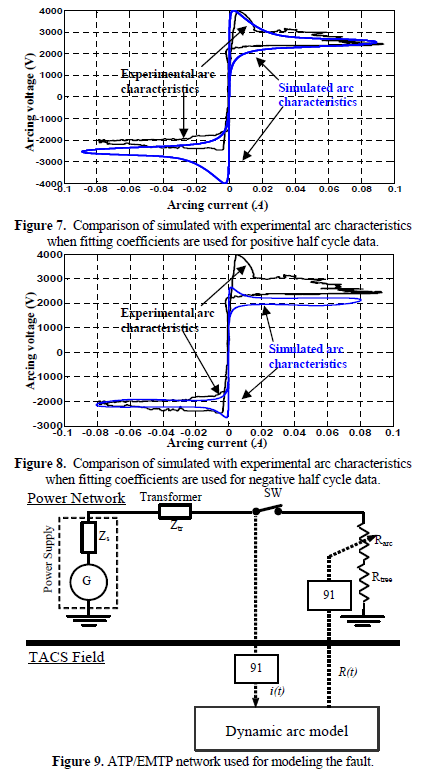## 4. Simulation Results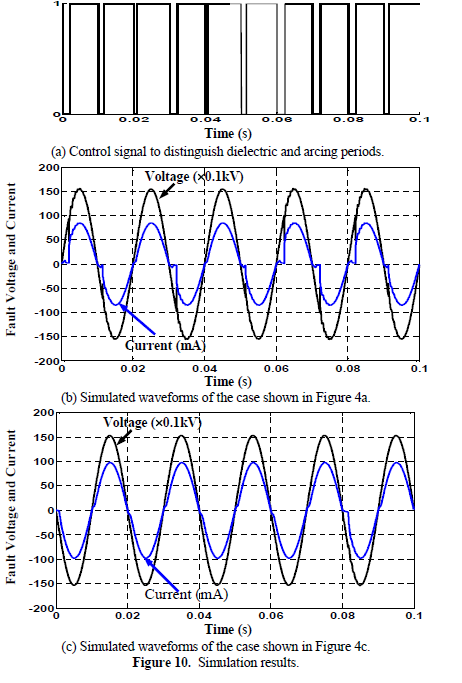## 5. DWT-Based fault features extraction

$D W T _ { \psi } {f ( m , k )} = \frac { 1 } { \sqrt { a _ { o } ^ { m } } } \sum _ { n } x ( n ) \psi ( \frac { k - n b _ { o } a _ { o } ^ { m } } { a _ { 0 } ^ { m } } ) \tag{4}$

### 5.1 Comparision of the fault features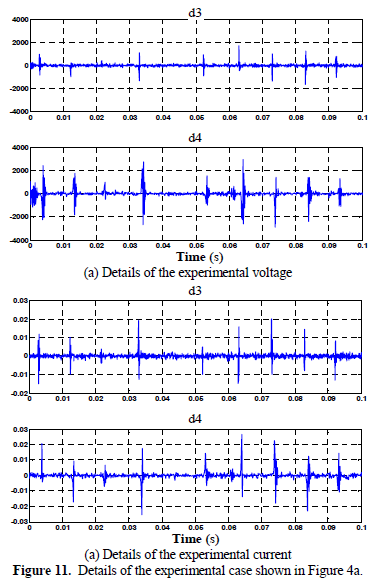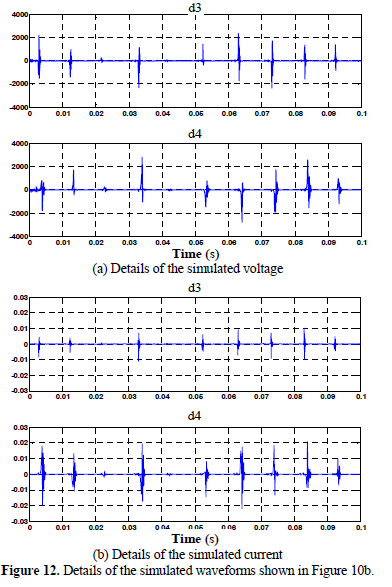### 5.2 Fault detection

$u_r=u_a+u_b+u_c\tag{5}$ $i_r=i_a+i_b+i_c\tag{6}$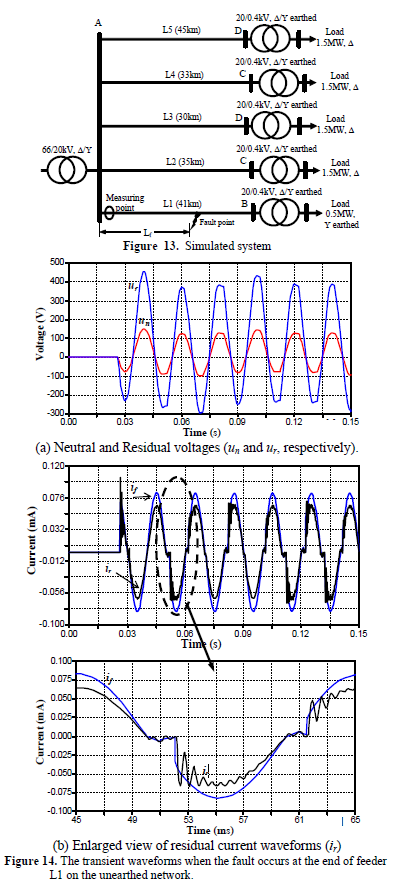Daubechies小波14（db14）还用于提取图14所示的故障情况的特征，其中考虑了100kHz的采样频率。但是，DWT执行时间是限制其实际实现的限制问题之一。这个问题正处于克服使用DSP板实验性地实现DWT的阶段，减少了其冗长的执行时间，如中所述。采样率可以降低;但是，应该使用不同的母小波。相应的细节如图16所示。很明显，由于电弧再燃，DWT对初始瞬态的响应经常出现在每个零交叉处。这证实了故障特征不仅在故障事件的起始时刻突出显示，而且在故障期间也突出显示。因此，电弧再燃的周期性是与倾斜树相关的故障相关的重要行为特征。因此，DWT性能可确保故障检测。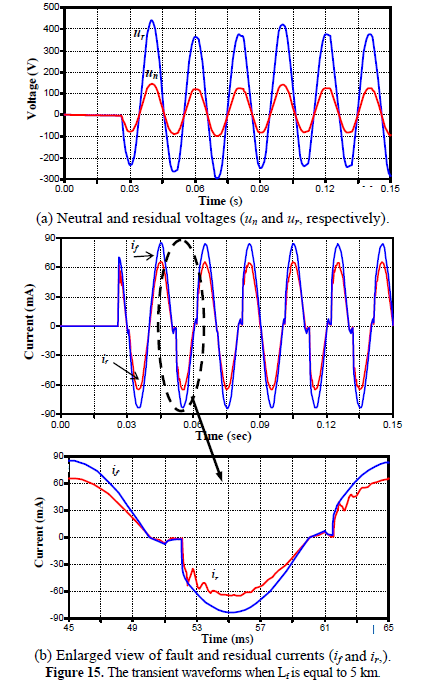## 6. Conclusions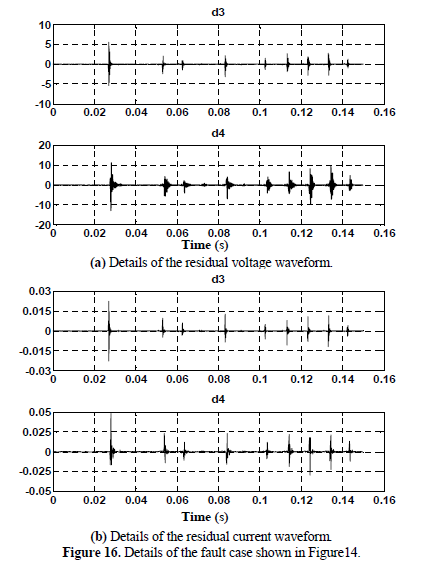## 7. Appendix